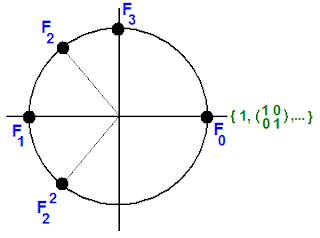occasional meanderings in physics' brave new world

## Saturday, October 25, 2008

### The MUB Tower

Given the collection of Fourier transforms $\{ F_{N} \}$ in all classical dimensions $N$, we note that they behave as $(N+1)$ roots of unity! Instead of the point $\omega$ on the complex plane, we put the Pauli MUB $F_{2}$ which cubes to unity.Since all $F_{N}$ satisfy $F_{N}^{N+1} = 1$, the unit matrices all live over the point 1 in the plane. In fact, there are $\mathbb{N}$ Fourier points over every rational point on the circle. Over the point $\omega$, for example, we can include all $F_{N}^{i}$ such that $\frac{N+1}{i} = 3$.

As any homotopy theorist knows, infinite covers of a circle are like (positive) real numbers. Only the numbers of the MUB Tower are q-numbers, which don't necessarily commute. All these numbers act on MUB operators to cycle them. This is a canonical way of turning the ordinals $\mathbb{N}$ (set cardinality) into a sequence of dimensions (vector space cardinality). The MUB Tower therefore naturally lives in the arithmetic of a quantum topos. We could try building a Hamiltonian from it. Hmmm. Now where have we heard that before?Matti Pitkänen said...

N-2:th roots of unity appear also in the definition of structure constants of elementary N-dimensional symplectic fusion algebra from which more complex fusion algebras are generated by replacing any element by another elementary fusion algebra. This gives operad whose algebras are labelled by arbitrary trees.

If the structure constant Cklm is non-vanishing it decomposes into a product

U_kU_lU_m

where each phase factor is N_2:th root of unity. That the sum of these roots vanishes is essential for the realization of associativity conditions.

In geometric realization the factors U_m correspond to non-integrable phase factors int A_mudx^mu assignable to the edges of symplectic triangles and one obtains fractional quantization symplecto-magnetic flux (or area quantization or quantization of solid angle).

October 25, 2008 8:29 PMKea said...

Excellent, Matti. I'm glad you can see such a fundamental use for MUB algebras. I'm sure there are many! I would like to think more about the symplectic geometry, but I'm afraid I have to dig the cafe out of the snow this morning.

October 26, 2008 7:43 AM